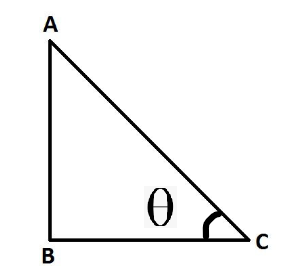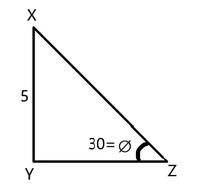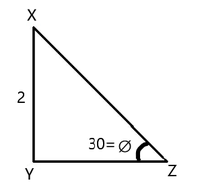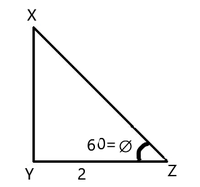# In a triangle XYZ right angled at Y, find the side length of YZ, if XY = 5 cm and ∠C = 30°

• Last Updated : 28 Jan, 2022

Trigonometry is the branch of mathematics that deals with triangles and angles associated with it. If the word is broken into 2 parts namely trigon and geometry, it can be understood easily that this branch of mathematics deals with the geometry of triangles. Using trigonometry, the properties of triangles and their applications can be easily understood. Using trigonometry, one can be finding the angles and missing sides of any triangle with the use of trigonometric ratios.

### Trigonometric Ratios

There are six functions or trigonometric ratios of an angle that are present in trigonometry. Their names and abbreviations are sine (sin), cosine (cos), tangent (tan), cotangent (cot), secant (sec), and cosecant (csc). One important thing to note here is that trigonometric formulas work only on the right-angle triangle. Let’s see the below image.In this right-angle triangle,  side AC is known as hypotenuse. Side BC is known as the base of the triangle. side AB is known as the height of the triangle. From the above triangle, we can list out formulas as above,

• sin∅ = AB/AC
• cos∅ = BC/AC
• tan∅ = AB/BC
• cot∅ = BC/AB
• cosec∅ = AC/AB
• sec∅ = AC/BC

Basic formulas of trigonometric ratios

• Tan ∅ = sin ∅/cos ∅
• sin ∅ = 1/cosec ∅
• cos ∅ = 1/sec ∅
• Tan ∅ = 1/cot ∅

### In a triangle XYZ right angled at Y, find the side length of YZ, if XY = 5 cm and ∠C = 30°

Solution:

Let’s understand this problem with the help of the below figure,What is needed to find is the base of the triangle YZ. The data which is given is angle Z is 30 and XY = 5

tan ∅ = XY/YZ

tan30 = 5/YZ

YZ = 5√3 (since tan30 = 1/√3)

### Similar Problems

Question 1: In a triangle XYZ, which is right-angled at Y, find the side length of XZ, if XY = 2 cm and ∠Z = 30°Solution:

Given, XY = 2 cm and ∠Z = 30°

To find – XZ

sin∅ = XY/XZ

sin30 = 2/XZ

XZ = 2/sin30

= 4 (since sin30° is 1/2)

Question 2: In a triangle XYZ, which is right-angled at Y, find the side length of XY, if YZ = 2 cm and ∠Z = 60°Solution:

Given, YZ = 2 cm and ∠Z = 60°

To find –  XY?

tan∅ = XY/YZ

tan60 = XY/2

XY = 2√3 (since tan60° is √3)

My Personal Notes arrow_drop_up